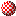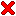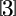#Subdivision Algorithms and AnalysisSubdivision is a technique in computer aided geometric design for the approximation of a smooth surface by a sequence of increasingly faceted polyhedra. Subdivision schemes have several attributes that have motivated their development since the work of Catmull-Clark '78, Doo-Sabin '78, and Loop '87:

• The input to the algorithm is a coarse mesh, that is manageable in size for the designer.
• The subdivision procedure on the mesh is determined by a simple set of affine combinations of the vertices.
• Several iterations typically result in a smooth surface.

Subdivision algorithms find their main application in industrial design and computer animations, for instance to model the skin of a human character. Pixar has used Catmull-Clark surfaces for 3d modeling in all their animation films since 1997.

Ulrich Reif introduced me to subdivision algorithms and the world of research in general, when I was a student at the Technische Universität Darmstadt/Germany until 2003. He proposed me to the Studienstiftung that subsequently supported my studies financially. He suggested to me to study overseas, and Joe Warren and Scott Schaefer mentored me as their research student at Rice University/United States.

The works listed below are the results of our collaboration:Volumetric subdivision schemes: While a large variety of subdivision algorithms exist to generate smooth surfaces, less attention has been given to volumetric subdivision schemes. In my thesis, I derive stationary subdivision rules on bi-uniform volumetric meshes consisting of pairwise combinations of tetrahedra, octahedra, triangular prisms, and cubes. The existing framework of quasi-interpolants is adapted so that weight stencils can be computed by algebraic manipulation. The joint spectral radius test developed by Adi Levin and David Levin in 2003 proves that the combined schemes yield C2 limit functions. The original idea to blend volumetric schemes is by Joe Warren.

Applications: Because subdivided volumetric meshes give a smooth parameterization of a region in 3d space, the method allows to generate character animations, see examples.Volume enclosed by subdivision surfaces: We derive the formula for the exact volume enclosed by subdivision surfaces. The formula requires the determination of a collection of trilinear forms. The trilinear forms depend on the subdivision weights, and the valence of a vertex of a quad, or triangular facet. To show the applicability of the formula in practice, we derive the forms for Catmull-Clark, Doo-Sabin, and Loop schemes and yield the volume of several example subdivision surfaces. We also generalize the formula for moments of arbitrary degree to include centroid and inertia defined by subdivision hyper-surfaces. Ulrich Reif introduced me to the problem already in 2002.

Applications: In the past, only an approximation of volume, centroid and inertia was available. Using the exact values instead increases realism of animations.

Our articles related to moments of sets bounded by subdivision manifolds are lined up here for quick access:

The preprint is published as an article in Applied Mathematics and Computation. The article is featured in Volume 272, Part 1: Subdivision, Geometric and Algebraic Methods, Isogeometric Analysis and Refinability and available for free, or for purchase in \$.Non-linear subdivision: Below, you can find a brief video on non-linear subdivision for curves on Riemannian manifolds.

There's no play here. There's no angle. There's no champagne room.
I'm not a miracle worker, I'm a janitor.
The math on this is simple.
The smaller the mess the easier it is for me to clean up.
Michael Clayton

##Loop, tri-quad, and tetrahedral subdivision in MATLAB

In collaboration with Annika Kuhl from Curtin University of Technology in Perth/Australia, we release a function to subdivide triangular meshes in MATLAB. The applied algorithms are Loop-subdision, but also linear subdivision.

Later, we also added subdivision of triangular and quadrilateral meshes. The algorithm is the extention of Loop- and Catmull-Clark-subdision described in Schaefer/Warren.

Upon a request by Jiayi Zhu from Zhejiang University in Hangzhou/China, a function to subdivide meshes consisting of tetrahedra and octahedra in MATLAB was added. The applied algorithms are Schaefer/Warren.

 Loop, tri-quad, and tetrahedral subdivision in MATLAB *mexmesh.zip 800 kB
* all source code included. The mex-binary is precompiled with Microsoft VC++ 6.0 for Windows; works for MATLAB 7.1 and later. However, according to Dylan Richard Muir, the cpp code does not compile on a Macintosh.
Weniges, aber Reifes.
Carl Friedrich Gauß

Below, you find a simple MATLAB example that generates a random triangular mesh and applies three rounds of Loop subdivision. The precompiled binary file subdivision.mexw32 in the zip-archive supplies the function subdivision(). Upon execution, a figure compares the input and output meshes.

```function loop_demo

X=rand(3,40);
T=delaunay(X(1,:),X(2,:));
T=T';

subplot(1,2,1);
trimesh(T',X(1,:),X(2,:),X(3,:))

[x,t]=subdivision(X,T,[0 1 1 1]);

subplot(1,2,2);
trimesh(t',x(1,:),x(2,:),x(3,:))
```

The actions performed by the function subdivision() are determined by a queue comprising of the digits:

• 0 - add mesh boundary
• 1 - one round of Loop-, tri-quad, or tetrahedral subdivision
• 2 - one round of linear subdivision

In the example, we use the sequence [0 1 1 1]: Boundary is added to the mesh, and three rounds of Loop-subdivision are applied. For precise information on the inputs and outputs to the function, please have a look at the demos provided in the software archive above.

When applying subdivision on an open mesh, we recommend to preceed subdivision by the "add boundary"-option. The option adds a curve along the border of a surface mesh, and a surface on the border of a valumetric mesh. Curves are subdivided using cubic B-spline subdivision. The measure prevents that the following rounds of subdivision deform the boundary of the mesh in an undesired fashion.

Zi Gong fragte: Gibt es ein Wort, nach dem man
sein ganzes Leben ausrichten kann?
Der Meister sprach: Ja. Gegenseitigkeit.
Konfuzius